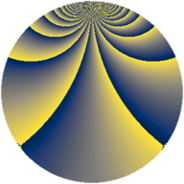# Properties

 Label 950.2.xLevel $950$ Weight $2$ Character orbit 950.x Rep. character $\chi_{950}(159,\cdot)$ Character field $\Q(\zeta_{30})$ Dimension $400$ Newform subspaces $1$ Sturm bound $300$ Trace bound $0$

# Related objects

## Defining parameters

 Level: $$N$$ $$=$$ $$950 = 2 \cdot 5^{2} \cdot 19$$ Weight: $$k$$ $$=$$ $$2$$ Character orbit: $$[\chi]$$ $$=$$ 950.x (of order $$30$$ and degree $$8$$) Character conductor: $$\operatorname{cond}(\chi)$$ $$=$$ $$475$$ Character field: $$\Q(\zeta_{30})$$ Newform subspaces: $$1$$ Sturm bound: $$300$$ Trace bound: $$0$$

## Dimensions

The following table gives the dimensions of various subspaces of $$M_{2}(950, [\chi])$$.

Total New Old
Modular forms 1232 400 832
Cusp forms 1168 400 768
Eisenstein series 64 0 64

## Trace form

 $$400q - 50q^{4} + 2q^{5} - 50q^{9} + O(q^{10})$$ $$400q - 50q^{4} + 2q^{5} - 50q^{9} - 12q^{11} + 8q^{14} - 18q^{15} + 50q^{16} - 10q^{17} - 12q^{19} + 4q^{20} - 32q^{21} + 20q^{22} + 30q^{23} - 14q^{25} + 60q^{27} + 24q^{29} - 52q^{30} + 20q^{33} + 8q^{34} - 8q^{35} + 50q^{36} - 24q^{39} - 16q^{41} + 4q^{44} - 144q^{45} - 32q^{46} + 120q^{47} - 440q^{49} - 40q^{50} + 52q^{51} + 40q^{53} - 12q^{54} - 88q^{55} + 16q^{56} + 48q^{59} - 2q^{60} - 28q^{61} + 10q^{63} + 100q^{64} + 152q^{65} + 16q^{66} - 80q^{67} - 16q^{69} + 8q^{70} - 14q^{71} + 80q^{73} - 104q^{75} - 8q^{76} - 80q^{77} + 60q^{78} - 8q^{79} + 2q^{80} + 106q^{81} + 80q^{83} + 56q^{84} + 78q^{85} - 20q^{86} + 80q^{87} + 12q^{89} + 78q^{90} - 8q^{91} - 20q^{92} + 4q^{95} - 30q^{97} - 40q^{98} - 12q^{99} + O(q^{100})$$

## Decomposition of $$S_{2}^{\mathrm{new}}(950, [\chi])$$ into newform subspaces

Label Dim. $$A$$ Field CM Traces $q$-expansion
$$a_2$$ $$a_3$$ $$a_5$$ $$a_7$$
950.2.x.a $$400$$ $$7.586$$ None $$0$$ $$0$$ $$2$$ $$0$$

## Decomposition of $$S_{2}^{\mathrm{old}}(950, [\chi])$$ into lower level spaces

$$S_{2}^{\mathrm{old}}(950, [\chi]) \cong$$ $$S_{2}^{\mathrm{new}}(475, [\chi])$$$$^{\oplus 2}$$